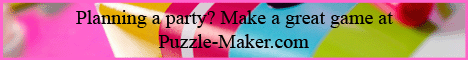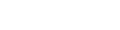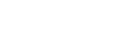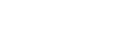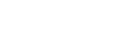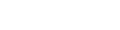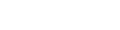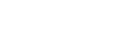Math Square 2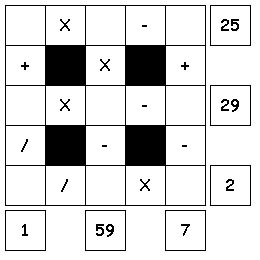Try to fill in the missing numbers. Use the numbers 1 through 9 to complete the equations. Each number is only used once. Each row is a math equation. Work from left to right. Each column is a math equation. Work from top to bottom. Click for solution.